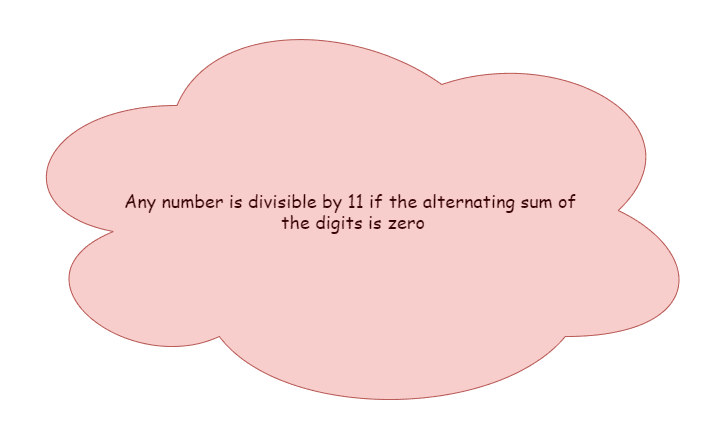Categories

# Divisibility AMC 8, 2017 problem 7

Try this beautiful problem from AMC 10. It involves geometry of triangles. We provide sequential hints so that you can try the problem.

## What are we learning ?

Competency in Focus: Divisibility This problem from American Mathematics contest (AMC 8, 2017) is based on the concept of divisibility .

## First look at the knowledge graph.## Next understand the problem

Let $Z$ be a 6-digit positive integer, such as 247247, whose first three digits are the same as its last three digits taken in the same order. Which of the following numbers must also be a factor of $Z$? $(A)11\qquad(B)19\qquad(A)101\qquad(A)111\qquad(A)1111$
##### Source of the problem
American Mathematical Contest 2017, AMC 8  Problem 7
Divisibility
2/10
##### Suggested Book

Do you really need a hint? Try it first!
Take $Z$ as $\overline{\rm ABCABC}$.
Calculate the alternating sum of the digits. i.e., $A-B+C-A+B-C=0$ then it is dibisible by $11$

# Connected Program at Cheenta

#### Amc 8 Master class

Cheenta AMC Training Camp consists of live group and one on one classes, 24/7 doubt clearing and continuous problem solving streams.

# Similar Problems

This site uses Akismet to reduce spam. Learn how your comment data is processed.